# 【知识点串讲】CM1 Survival models 生存模型

CM1寿险精算部分的第一课。

## 余命的随机变量

### 符号标记和定义

$$(x)$$ 表示当前为 $$x$$ 岁的个体，我们用 $$T_{x}$$$$K_{x}$$ 表示余命的随机变量：

• $$T_{x}$$ 表示 $$(x)$$ 的余命的随机变量（Future lifetime random variable），特别地，$$T_{0}$$ 为新生儿（newborn）的余命的随机变量；
• $$K_{x}$$ 表示 $$(x)$$ 的取整余命的随机变量(Curtate future lifetime random variable)，$$K_{x}$$$$T_{x}$$ 的整数部分，即：$$K_{x}=[ T_{x}]$$.

• $$T_{x}$$ 的累积分布函数（Cumulative Distribution Function, cdf）：$$F_{x}(t)=P(T_{x}\leq t)$$

• $$T_{x}$$ 的生存函数（Survival Function, sf）：$$S_{x}(t)=1-F_{x}(t)=P(T_{x}\geq t)$$

• $$T_{x}$$ 的概率密度函数（Probability Density function, pdf）：$$f_{x}(t)=\dfrac{d}{dt}F_{x}(t)$$

## $$T_{0}$$的分布

• cdf of $$T_{0}$$: $$F_{0}(x)=P(T_{0}\leq x)$$

• sf of $$T_{0}$$: $$S_{0}(x)=P(T_{0}> x)=1-F_{0}(x)$$

• pdf of $$T_{0}$$: $$f_{0}(x)=\frac{d}{dx}F_{0}(x)=-\frac{d}{dx}S_{0}(x)$$

## $$T_{0}$$ 和 $$T_{x}$$ 的关系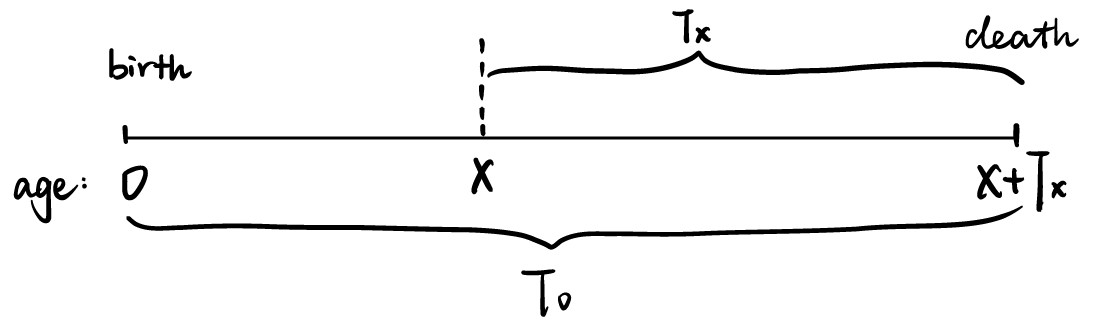## $$T_{x}$$的分布（用$$T_{0}$$的分布表示）

• cdf of $$T_{x}$$: $$F_{x}(t)=P(T_{x}\leq t)=\frac{F_{0}(x+t)-F_{0}(x)}{1-F_{0}(x)}$$

• sf of $$T_{x}$$: $$S_{x}(t)=P(T_{x}> t)=1-F_{x}(t)=\frac{S_{0}(x+t)}{S_{0}(x)}$$

• pdf of $$T_{x}$$: $$f_{x}(t)=\frac{d}{dt}F_{x}(t)=\frac{f_{0}(x+t)}{S_{0}(x)}$$

## Chaining Survival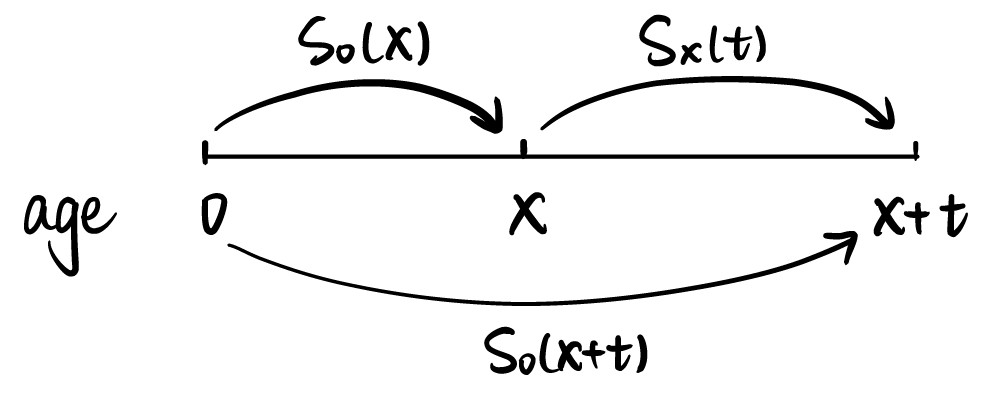$S_{0}(x+t)=S_{0}(x)S_{x}(t)$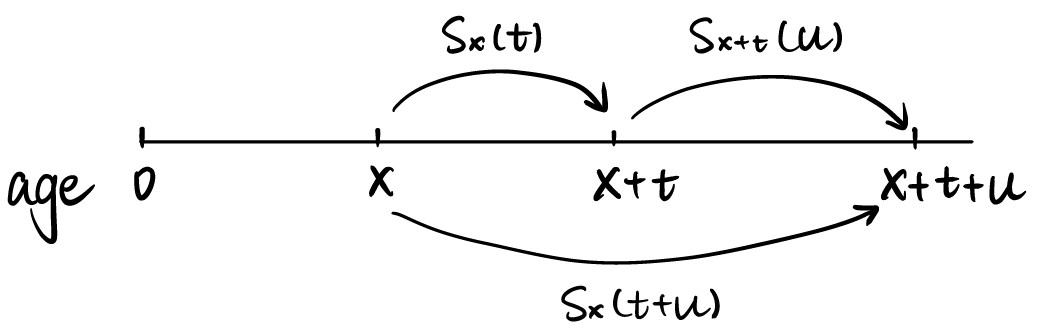$S_{x}(t+u)=S_{x}(t)S_{x+t}(u)$

## 精算标记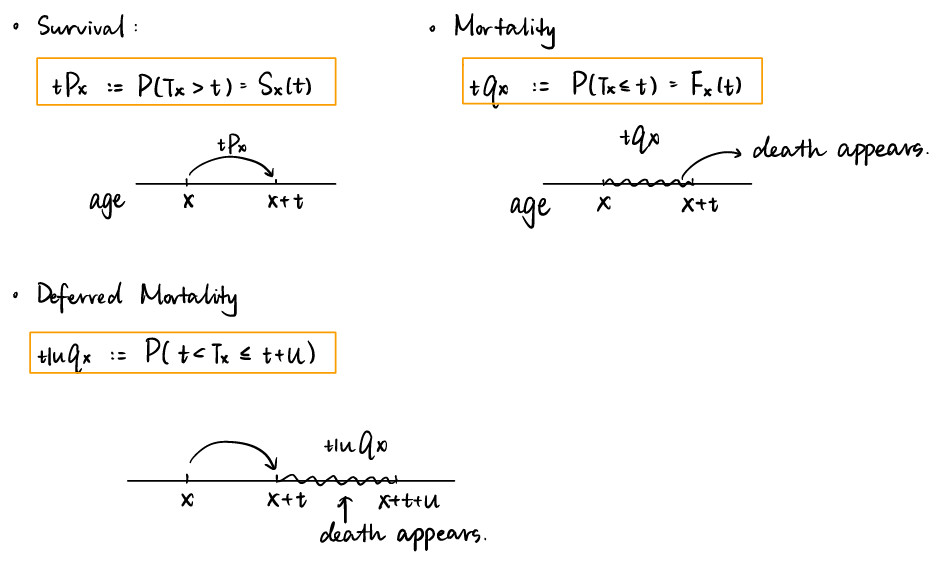• Survival: $$\px{t}{x}=P(T_{x}> t)=S_{x}(t)$$
• Mortality: $$\qx{t}{x}=P(T_{x}\leq t)=F_{x}(t)$$
• Deferred Mortality: $$\qx{t|u}{x}=P(t < T_{x}\leq t+u)$$

$$t=1$$ 时，符号中的1可以这样省略： $$\px{1}{x}=\px{}{x}$$, $$\qx{1}{x}=\qx{}{x}$$, $$\qx{t|1}{x}=\qx{t|}{x}$$.

• $$\px{t+u}{x}=\px{t}{x}\cdot \px{u}{x+t}=\px{u}{x}\cdot \px{t}{x+u}$$
• $$\qx{t+u}{x}=1-\px{t}{x} \times \px{t}{x+u}=1-(1- \qx{u}{x})(1- \qx{t}{x+u})$$

### 3种计算 $$\qx{t|u}{x}$$ 的方法

• $$\qx{t|u}{x}=F_{x}(t+u)-F_{x}(t)=\qx{t+u}{x}-\qx{t}{x}$$
• $$\qx{t|u}{x}=S_{x}(t)-S_{x}(t+u)=\px{t}{x}-\px{t+u}{x}$$
• $$\qx{t|u}{x}=\px{t}{x}\cdot \qx{u}{x+t}$$

### 分布函数 $$F$$ 和概率密度函数 $$f$$ 的关系

$\qx{t}{x}=\int_{0}^{t} \px{u}{x}\mu_{x+u}du$

## 死亡力

$$\mu_{x}$$，称为 force of mortality, hazard rate, transition intensity, 定义为： \begin{aligned} \mu_{x}&=\lim\limits_{dx\to 0^{+}}\frac{P(T_{0}\leq x+dx|T_{0}>x)}{dx}\\\\ &=\lim\limits_{dx\to 0^{+}}\frac{P(T_{x}\leq dx)}{dx} \end{aligned}

$$\mu_{x}$$可以理解为$$(x)$$在极短的时间$$dx$$内死亡的“极限概率”（注意，不是概率），或者说瞬时的死亡率。$$\mu_{x}$$是 rate，而不是 probability。

### 用 $$T_{0}$$ 的分布表示 $$\mu_{x}$$

$\mu_{x}=\dfrac{f_{0}(x)}{S_{0}(x)} =-\frac{1}{S_{0}(x)} \cdot \frac{d}{d_{x}} S_{0}(x) =-\frac{\partial}{\partial x} \log S_{0}(x)$

### 用 $$T_{x}$$ 的分布表示 $$\mu_{x+t}$$

$\mu_{x+t}=\frac{f_{x}(t)}{S_{x}(t)}=-\frac{\partial}{\partial x}log S_{x}(t)$

$\px{t}{x}=S_x(t)=exp{(-\int_{0}^{t}\mu_{x+r}dr)}$

### 用 $$\mu_{x+t}$$ 表示概率密度函数 $$f_{x}(t)$$

$f_{x}(t) =-\frac{d}{d t} S_{x}(t) =\mu_{x+t}\cdot \px{t}{x}$

## 典型的人类死亡力曲线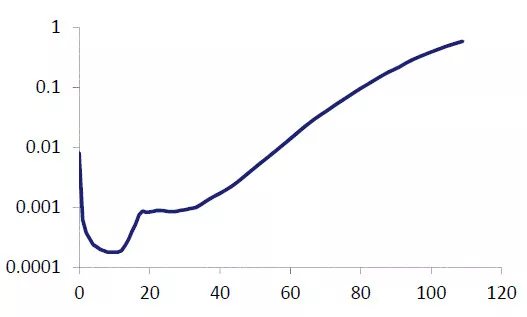$$\mu_{x}$$ on $$log_{10}$$ scale (ELT15 (Males) Mortality Table) The main features of $$\mu_{x}$$ are:

• High infant mortality
• An ‘accident hump’ at ages around 20
• The nearly exponential increase at older ages

## $$T_{x}$$ 的分布特征

### Median of $$T_{x}$$

$$T_{x}$$的中位数（Median）为使得 $$P(T_{x}>m)=0.5$$$$m$$，即： $$\px{m}{x}=0.5$$

### Variance of $$T_{x}$$

$$T_{x}$$的方差（Variance）： $Var[T_{x}]=E[T_{x}^{2}]-(E[T_{x}])^{2}$

## $$K_{x}$$ 的分布特征

$$K_{x}=[T_{x}]$$，也就是说，$$K_{x}$$代表complete/full years of future life for $$(x)$$的整数部分。

• cdf: $$P(K_{x}\leq k)=1-\px{k+1}{x}=\qx{k+1}{x}$$

• sf:$$P(K_{x}> k)=\px{k+1}{x}$$

### Variance of $$K_{x}$$

$Var[K_{x}]=2\sum_{k=1}^{\infty}k \cdot \px{k}{x}-e_{x}-(e_{x})^{2}$

### $$e_{x}$$ 和 $$\mathring{e}_x$$ 的联系

$\mathring{e}_x \approx e_x+\frac{1}{2}$